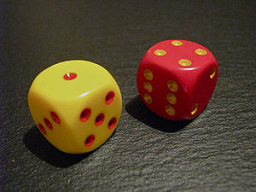# Probabilities 71194

We have a dummy die where numbers fall with probabilities P (1)=0.1; P (2)=0.2; P (3)=0.22; P (4)=0.16; P (5)=0.24; P (6)=0.08. Determine the probability that the two tosses the same numbers.

p =  0.188

### Step-by-step explanation:Did you find an error or inaccuracy? Feel free to write us. Thank you!

Showing 1 comment:
Zee
I like this so much!

Tips for related online calculators
Need help calculating sum, simplifying, or multiplying fractions? Try our fraction calculator.
Would you like to compute the count of combinations?